# Paraconsistent logic

(diff) ← Older revision | Latest revision (diff) | Newer revision → (diff)

A relation of logical consequence,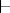, on a set of sentences,, is explosive if and only if for all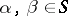,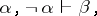where "¬" is negation. A relation, and the logic that possesses it, is paraconsistent if and only if it is not explosive. Whether or not a correct consequence relation is explosive has been a contentious issue historically, but the standard formal logics of the 20th century, such as classical logic (cf. Logical calculus) and intuitionistic logic are explosive. Formal paraconsistent logics were developed by a number of different people, often working in isolation from each other, starting around the 1960s.

There are many different paraconsistent logics, with their own proof theories and model theories. Their distinctive features occur at the propositional level, though they all have full first- (and second-) order versions. In most of them validity can be defined in terms of preservation of truth in an interpretation.

In one approach, due to S. Jaśkowski, an interpretation is a Kripke model (cf. Kripke models) for some modal logic, and a sentence is true in it if it holds at some world of the interpretation. A major feature of this approach is that the inference of adjunction (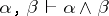) fails. In another, an interpretation,{} is a mapping fromto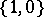, satisfying the usual classical conditions for,, and.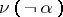is independent of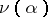. The addition of further constraints on, such as: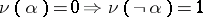, give logics in N. da Costa'sfamily. A feature of this approach is that it preserves all of positive logic. In a third approach, an interpretationis a mapping fromto the closed sets of a topological space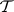satisfying the conditions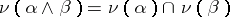,,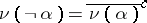(whereis the closure operator of).is true underif and only ifis the whole space. This gives a logic dual to intuitionistic logic.

In a fourth approach, an interpretation is a relation, satisfying the natural conditions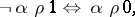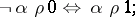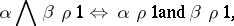and dually for.is true underif and only if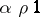. This gives the logic of first degree entailment (FDE) of A. Anderson and N. Belnap. If one restricts interpretations to those satisfying the condition, one gets G. Priest's LP. A feature of this logic is that its logical truths coincide with those of classical logic. Thus, the law of non-contradiction holds: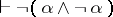. A De Morgan lattice is a distributive lattice with an additional operatorsatisfying: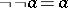and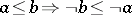. An FDE-interpretation can be thought of as a homomorphism into the De Morgan lattice with values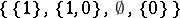. More generally,in FDE if and only if for every homomorphisminto a De Morgan lattice,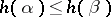. Augmenting such lattices with an operatorsatisfying certain conditions, and defining validity in the same way, gives a family of relevant logics.

A paraconsistent logic localizes contradictions, and so is appropriate for reasoning from information that may be inconsistent, e.g., information stored in a computer database. It also permits the existence of theories (sets of sentences closed under deducibility) that are inconsistent but not trivial (i.e., containing everything) and of their models, inconsistent structures.

One important example of an inconsistent theory is set theory based on the general comprehension schema (, whereis any formula not containing), together with extensionality (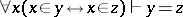). Another is a theory of truth (or of other semantic notions), based on the-schema (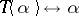, whereis any closed formula, andindicates a name-forming device), together with some mechanism for self-reference, such as arithmetization. Such theories are inconsistent due to the paradoxes of self-reference (cf. Antinomy).

Not all paraconsistent logics are suitable as the underlying logics of these theories. In particular, if the underlying logic contains contraction () and modus ponens (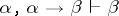), these theories are trivial. However, the theories are non-trivial ifis interpreted as the material conditional and the logic LP is used, or if it is interpreted as the conditional of some relevant logics. In the truth theories, the inconsistencies do not spread into the arithmetical machinery.

Given a topos, logical operators can be defined as functors within it, and a notion of internal validity can be defined, giving intuitionistic logic. If these operators, and in particular, negation, are defined in the dual way, the internal logic of the topos is the dual intuitionistic logic. Topoi can therefore be seen as inconsistent structures.

For another example of inconsistent structures, letbe the set of sentences true in the standard model of arithmetic. Ifis a set of sentences in the same language properly containing, thenis inconsistent, and so has no classical models; buthas models, including finite models, in the paraconsistent logic LP. Inconsistent (sets of) equations may have solutions in such models. The LP-models ofinclude the classical non-standard models of arithmetic (cf. Peano axioms) as a special case, and, like them, have a notable common structure.

In inconsistent theories of arithmetic, the incompleteness theorems of K. Gödel (cf. Gödel incompleteness theorem) fail: such a theory may be axiomatizable and contain its own "undecidable" sentence (and its negation).

Inconsistent theories may be interesting or useful even if they are not true. The view that some inconsistent theories are true is called dialetheism (or dialethism).

For a general overview of the area, see [a2]. [a3] is a collection of articles, with much background material. On inconsistent mathematical structures, see [a1].

How to Cite This Entry:
Paraconsistent logic. Encyclopedia of Mathematics. URL: http://encyclopediaofmath.org/index.php?title=Paraconsistent_logic&oldid=17427
This article was adapted from an original article by G. Priest (originator), which appeared in Encyclopedia of Mathematics - ISBN 1402006098. See original article